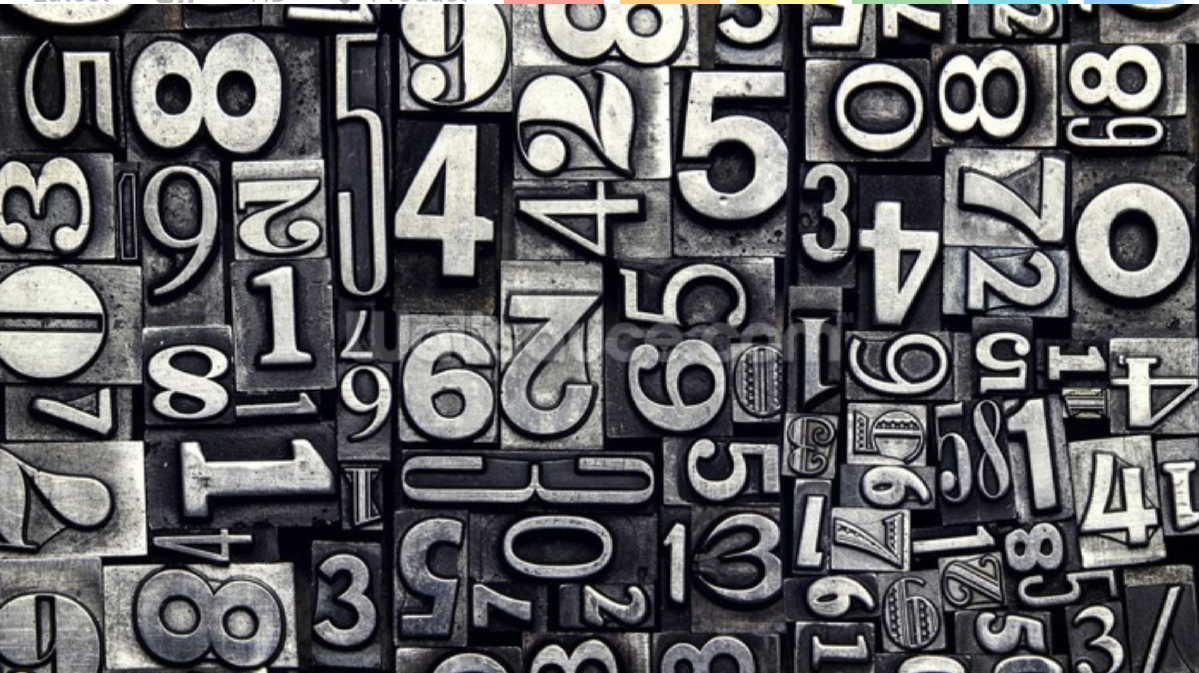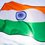# Favourite numbers

The number should have some interesting property which makes it your favourite.

Note : your number should be a natural number.Note by Mr. India
2 years, 3 months ago

This discussion board is a place to discuss our Daily Challenges and the math and science related to those challenges. Explanations are more than just a solution — they should explain the steps and thinking strategies that you used to obtain the solution. Comments should further the discussion of math and science.

When posting on Brilliant:

• Use the emojis to react to an explanation, whether you're congratulating a job well done , or just really confused .
• Ask specific questions about the challenge or the steps in somebody's explanation. Well-posed questions can add a lot to the discussion, but posting "I don't understand!" doesn't help anyone.
• Try to contribute something new to the discussion, whether it is an extension, generalization or other idea related to the challenge.

MarkdownAppears as
*italics* or _italics_ italics
**bold** or __bold__ bold
- bulleted- list
• bulleted
• list
1. numbered2. list
1. numbered
2. list
Note: you must add a full line of space before and after lists for them to show up correctly
paragraph 1paragraph 2

paragraph 1

paragraph 2

[example link](https://brilliant.org)example link
> This is a quote
This is a quote
    # I indented these lines
# 4 spaces, and now they show
# up as a code block.

print "hello world"
# I indented these lines
# 4 spaces, and now they show
# up as a code block.

print "hello world"
MathAppears as
Remember to wrap math in $$ ... $$ or $ ... $ to ensure proper formatting.
2 \times 3 $2 \times 3$
2^{34} $2^{34}$
a_{i-1} $a_{i-1}$
\frac{2}{3} $\frac{2}{3}$
\sqrt{2} $\sqrt{2}$
\sum_{i=1}^3 $\sum_{i=1}^3$
\sin \theta $\sin \theta$
\boxed{123} $\boxed{123}$

Sort by:

My favourite number is $142857$

It is a cyclic number

It is a kaprekar number

$\frac{1}{7}=0.\overline{142857}$ and interestingly, $\frac{1}{142857}=0.\overline{000007}$

$142+857=999$ and $14+28+57=99$

- 2 years, 3 months ago

My favorite number is $4679307774$. It has $10$ digits, and it you sum each digit raised to the $10$th power (e.g. $4^{10}+6^{10}+7^{10}+9^{10}+3^{10}+0^{10}+7^{10}+7^{10}+7^{10}+4^{10}$), you will get $4679307774$, the same number.

- 2 years, 3 months ago

Interesting!!

- 2 years, 3 months ago

Are there any other numbers with similar property?

- 2 years, 3 months ago

Yes. View this oeis page.

- 2 years, 3 months ago

Does such a number exists in every base ? So in base n a n-digit number with is the same as raise the digits to n and add up these numbers.

- 2 years, 3 months ago

That is a really interesting question, to which I have no answer. I would definitely love to look into this more, though.

- 2 years, 2 months ago

- 2 years, 2 months ago

Thanks so much!!

- 2 years, 2 months ago

73 and 42.

73 is the 21th prime number

$21 = 7 * 3$

37 is the 12th prime number(Do you see the symmetry).

73 is in base 2 1001001 which is symmetric.

42 is coolest of each number(hopefully you know why, if you don't know why, don't panic ).

- 2 years, 3 months ago

Nice symmetry. And yes, 42 is the supreme number. Don't forget your towel!

- 2 years, 3 months ago

42 is so fly, I accidentally missed the ground

- 2 years, 3 months ago

- 2 years, 3 months ago

Read the "The Hitchhiker's Guide to the Galaxy" book and you will understand everything. Sometimes it is a litte bit confusion, but it is still a essential book for people who do something with math (why? I don't know).

In other words 42 is a random number, which a random guy wrote in a random book, and it is the answer of life, universe and everything, and that's why it is so special.

- 2 years, 3 months ago

42 is not a simple random number. There are a number of properties for which, it is the first or the last know number having them. And at least for one:

"42 is the only known value that is the number of sets of four distinct positive integers a, b, c, d, each less than the value itself, such that ab − cd, ac − bd, and ad − bc are each multiples of the value. Whether there are other values remains an open question."

Quoted from the Wikipedia.

- 2 years, 3 months ago

Cool properties... But for Douglas Adams was 42 still a random number.

- 2 years, 2 months ago

Actually I loved the bits of The Hitchhiker's Guide to the Galaxy, that I heard once on the radio. Forgot about 42 being the answer to everything. Must read it properly. Thanks.

- 2 years, 2 months ago

Reference from big bang theory? :)

- 2 years, 2 months ago

Yes ;)

- 2 years, 2 months ago

Don't laugh, I think my favorite number is 64. My life is full of coincidences related with that number, starting with my birthday year. 6+4=10 is the base of our decimal system, 64 is the 6th power of 2, 6 is the first perfect number and 4 is also a power of 2, 6-4=2, my favorite numerical base, 6*4=24, the number of hours of our day, so, overall, it has a lot of other of my favorite numbers. These is not over the top properties but it is enough to me.

- 2 years, 3 months ago

I never saw 64 that way.

- 2 years, 3 months ago

24 because it is 4!, (2^1)(3^2)(1^3), it is an antiprime, also because of challenge 24, 24 hours in a day, 2+2=4, 2*2=4, 2^2=4, 2^(24-1)=8388608 which is a pretty cool power of 2 since it contains a lot of 8's, and 5^2-1^2, 7^2-5^2.

- 1 year, 12 months ago

Pretty interesting!

- 1 year, 11 months ago

Well, my favourite number, even though is kind of cliche, is the Euler Mascheroni Constant!!

I mean it surprises me that we do not have its closed form!!! And this is the kind of number which can be EASILY explained to anyone using its definition, but yet, no one would know the vast amount of properties it has.....!!

- 2 years, 3 months ago

Curious number indeed!

- 2 years, 3 months ago

You forgot, it should have be a natural number. I'm not taking it down, I'm sure it must be a very sexy number.

- 2 years, 3 months ago

13, because no one like it

- 2 years, 3 months ago

Strange reason ;-)

- 2 years, 3 months ago

lol reverse psychology

- 2 years, 3 months ago